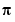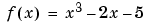MATLAB Function Referencefzero

Find zero of a function of one variable

Syntax

• ```x = fzero(fun,x0)
x = fzero(fun,x0,options)
x = fzero(fun,x0,options,P1,P2,...)
[x,fval] = fzero(...)
[x,fval,exitflag] = fzero(...)
[x,fval,exitflag,output] = fzero(...)
```

Description

```x = fzero(fun,x0) ``` tries to find a zero of `fun` near `x0`, if `x0` is a scalar. The value `x` returned by `fzero` is near a point where `fun` changes sign, or `NaN` if the search fails. In this case, the search terminates when the search interval is expanded until an `Inf`, `NaN`, or complex value is found.

If `x0` is a vector of length two, `fzero` assumes `x0` is an interval where the sign of `fun(x0(1))` differs from the sign of `fun(x0(2))`. An error occurs if this is not true. Calling `fzero` with such an interval guarantees `fzero` will return a value near a point where `fun` changes sign.

```x = fzero(fun,x0,options) ``` minimizes with the optimization parameters specified in the structure `options`. You can define these parameters using the `optimset` function. `fzero` uses these `options` structure fields:

 `Display` Level of display. `'off'` displays no output; `'iter'` displays output at each iteration; `'final'` displays just the final output; `'notify'` (default) dislays output only if the function does not converge. `TolX` Termination tolerance on `x.`

```x = fzero(fun,x0,options,P1,P2,...) ``` provides for additional arguments passed to the function, `fun`. Use `options = []` as a placeholder if no options are set.

```[x,fval] = fzero(...) ``` returns the value of the objective function `fun` at the solution `x`.

```[x,fval,exitflag] = fzero(...) ``` returns a value `exitflag` that describes the exit condition of `fzero`:

 `>0` Indicates that the function found a zero `x.` `<0` No interval was found with a sign change, or a `NaN` or `Inf` function value was encountered during search for an interval containing a sign change, or a complex function value was encountered during the search for an interval containing a sign change.

```[x,fval,exitflag,output] = fzero(...) ``` returns a structure `output` that contains information about the optimization:

 `output.algorithm` The algorithm used `output.funcCount` The number of function evaluations `output.iterations` The number of iterations taken

 Note    For the purposes of this command, zeros are considered to be points where the function actually crosses, not just touches, the x-axis.

Arguments

`fun` is the function whose zero is to be computed. It accepts a vector `x` and returns a scalar `f`, the objective function evaluated at `x`. The function `fun` can be specified as a function handle.

• ```x = fzero(@myfun,x0)
```

where `myfun` is a MATLAB function such as

• ```function f = myfun(x)
f = ...            % Compute function value at x
```

`fun` can also be an inline object.

• ```x = fzero(inline('sin(x*x)'),x0);
```

Other arguments are described in the syntax descriptions above.

Examples

Example 1. Calculateby finding the zero of the sine function near 3.

• ```x = fzero(`@`sin,3)
x =
3.1416
```

Example 2. To find the zero of cosine between 1 and 2

• ```x = fzero(`@`cos,[1 2])
x =
```

1.5708

Note that `cos(1)` and `cos(2)` differ in sign.

Example 3. To find a zero of the functionwrite an M-file called `f`.`m`.

• ```function y = f(x)
y = x.^3-`2`*`x-5`;
```

To find the zero near 2

• ```z = fzero(@f`,`2)
z =
2.0946
```

Because this function is a polynomial, the statement `roots([1 0 -2 -5])` finds the same real zero, and a complex conjugate pair of zeros.

• ```    2.0946
-1.0473 + 1.1359i
-1.0473 - 1.1359i
```

Algorithm

The `fzero` command is an M-file. The algorithm, which was originated by T. Dekker, uses a combination of bisection, secant, and inverse quadratic interpolation methods. An Algol 60 version, with some improvements, is given in . A Fortran version, upon which the `fzero` M-file is based, is in .

Limitations

The `fzero` command finds a point where the function changes sign. If the function is continuous, this is also a point where the function has a value near zero. If the function is not continuous, `fzero` may return values that are discontinuous points instead of zeros. For example, `fzero(@tan,1)` returns `1.5708`, a discontinuous point in `tan`.

Furthermore, the `fzero` command defines a zero as a point where the function crosses the x-axis. Points where the function touches, but does not cross, the x-axis are not valid zeros. For example, `y = x.^2` is a parabola that touches the x-axis at 0. Because the function never crosses the x-axis, however, no zero is found. For functions with no valid zeros, `fzero` executes until `Inf`, `NaN`, or a complex value is detected.

`roots`, `fminbnd`, `function_handle` (@), `inline`, `optimset`fwrite (serial) gallery